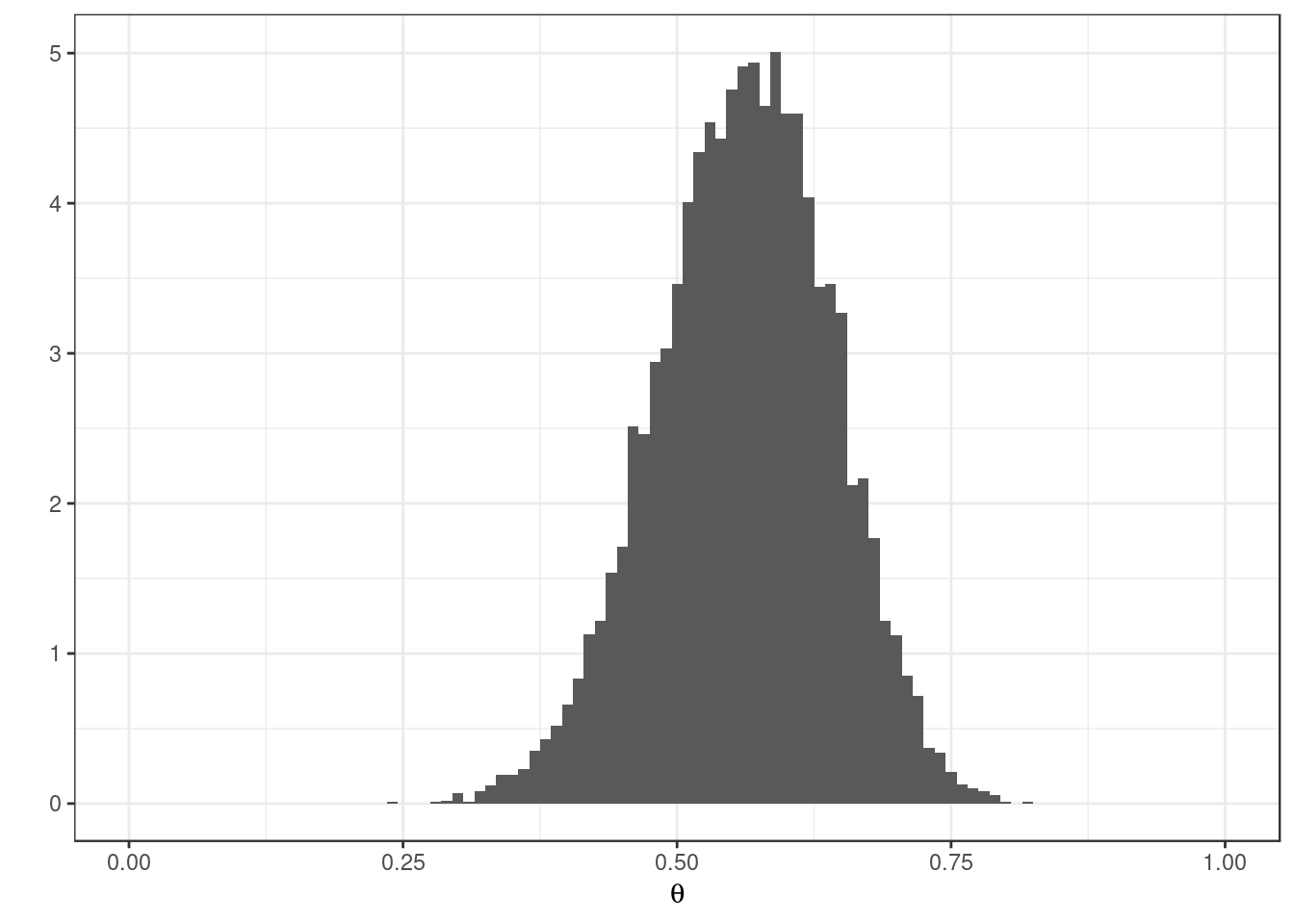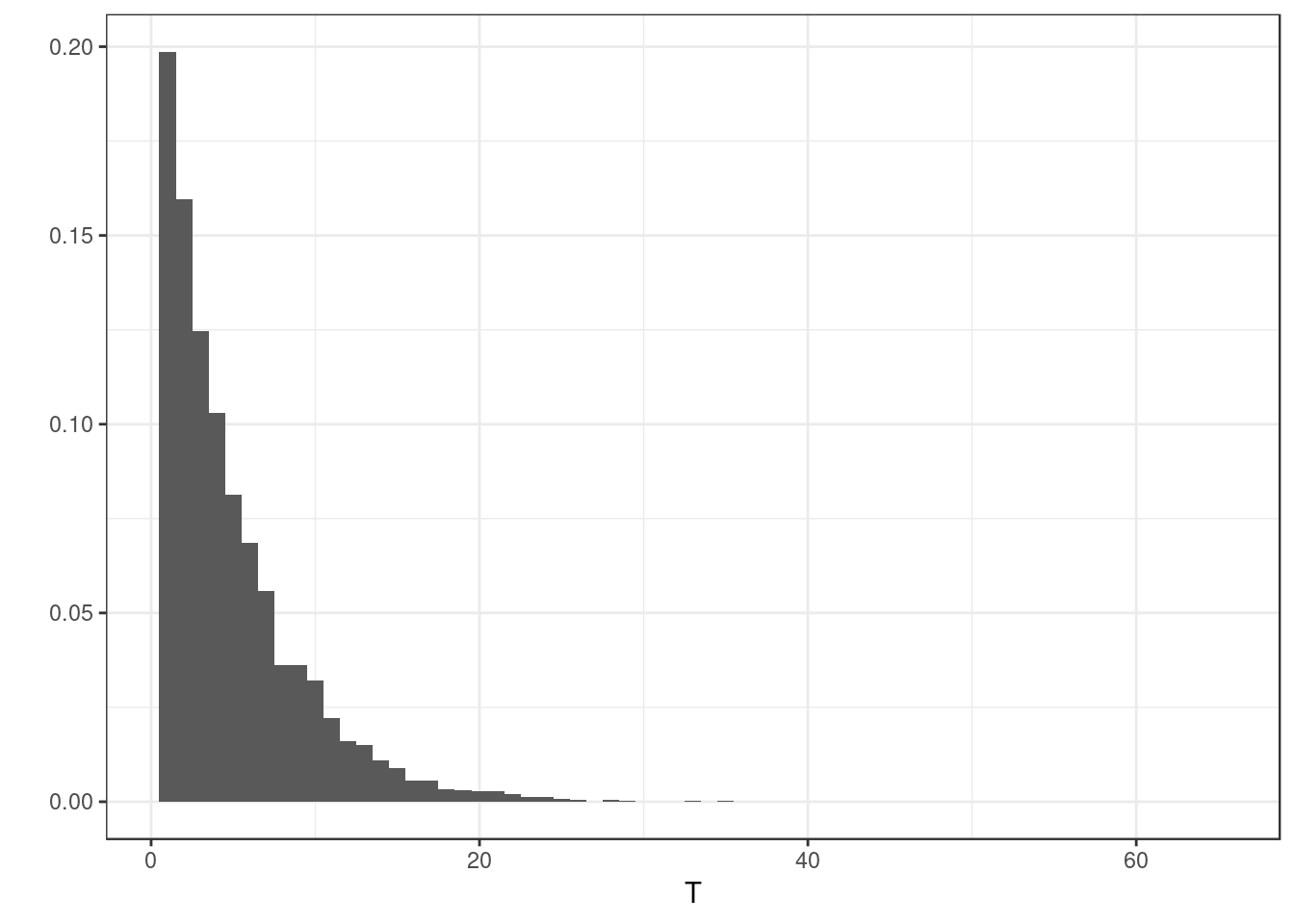This corresponds to the example found in Lecture 3.

First set the random seed to ensure this program’s output is the same every time it’s run. Any arbitrary number will do.

set.seed(17)

Generate synthetic data from the model.

true_theta <- 0.6

# A function to map from theta to the corresponding vector of outcome
# probabilities.
multinomial_probs <- function(theta) {
c(1/2 + theta/4, (1/4) * (1 - theta), (1/4) * (1 - theta), theta/4)
}

# Sample observations from the multinomial distribution.
y <- rmultinom(1, size = 100, multinomial_probs(true_theta))
print(y)
##      [,1]
## [1,]   69
## [2,]    9
## [3,]   11
## [4,]   11

Define the (log) likelihood function and find the MLE.

loglikelihood <- function(theta) {
# Use R's builtin function for multinomial likelihood.
dmultinom(y, prob = multinomial_probs(theta), log = TRUE)
}

# Maximize the log-likelihood by minimizing the negative log-likelihood.
o <- optimize(function(x) -loglikelihood(x), interval = c(0, 1))
mle <- o\$minimum
mle_loglikelihood <- loglikelihood(mle)
cat("mle: ", mle)
## mle:  0.5676134
cat("log likelihood at its maximum: ", mle_loglikelihood)
## log likelihood at its maximum:  -6.819816

Use the accept-reject algorithm to sample from the posterior corresponding to a uniform prior. Use a uniform distribution as the proposal.

nsamples <- 10000
naccepts <- 0
samples <- rep(0, nsamples)

# The following variables are only for analyzing the performance of this
# algorithm.
waitingtimes <- rep(0, nsamples)
ncandidates <- 0

# Run algorithm until we have the desired number of accepted samples.
while (naccepts < nsamples) {
ncandidates <- ncandidates + 1
waitingtimes[naccepts + 1] <- waitingtimes[naccepts + 1] + 1
x <- runif(1)
logalpha <- loglikelihood(x) - mle_loglikelihood
if (log(runif(1)) < logalpha) {
naccepts <- naccepts + 1
samples[naccepts] <- x
}
}
cat("number of accepted points: ", naccepts, "out of ", ncandidates, "candidates")
## number of accepted points:  10000 out of  49830 candidates

Plot the results.

library(ggplot2)
theme_set(theme_bw())
grejecthist <- qplot(x = samples, geom = "blank") + geom_histogram(aes(y = ..density..),
binwidth = 0.01) + xlab(expression(theta)) + scale_x_continuous(limits = c(0,
1))
grejecthist
## Warning: Removed 2 rows containing missing values (geom_bar).grejectwait <- qplot(x = waitingtimes, geom = "blank") + geom_histogram(aes(y = ..density..),
binwidth = 1) + xlab(expression(T))
grejectwait